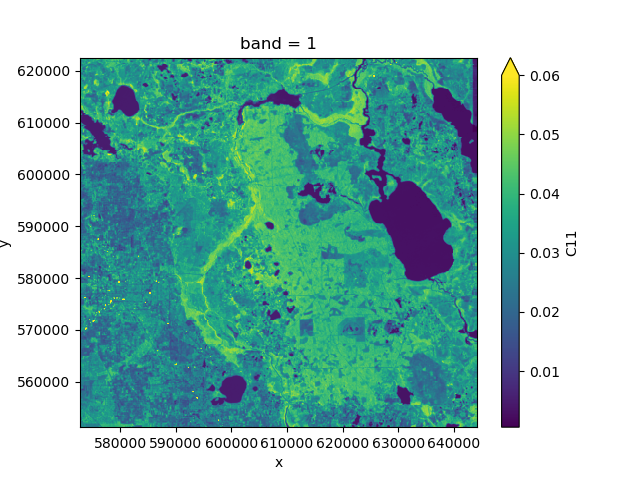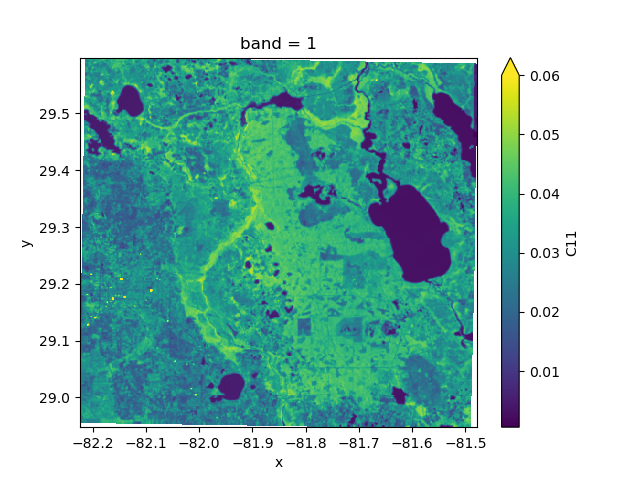# 5. Projections

`nd` handles geographic projections with `rasterio`. Projection information is usually stored in the metadata attributes `crs` and `transform`, but different standards exist.

All functionality related to coordinate systems and projections is contained in the module `nd.warp`. You can extract the coordinate reference system of a dataset using `nd.warp.get_crs()`:

```>>> from nd.warp import get_crs
>>> get_crs(ds)
CRS.from_epsg(3086)
```

The returned object is always of type `rasterio.crs.CRS`.

Similarly, the coordinate transformation can be extracted using `nd.warp.get_transform()`:

```>>> from nd.warp import get_transform
>>> get_transform(ds)
Affine(178.27973915722524, 0.0, 572867.3883891336,
0.0, -178.27973915722524, 622453.9641249835)
```

which is an `affine.Affine` object and represents the mapping from image coordinates to projection coordinates.

Additionally, a dataset contains the coordinates of the x and y axes in `ds.coords['x']` and `ds.coords['y']`. The transform object and the coordinate arrays represent the same information.

## 5.1. Reprojecting to a different CRS

You can reproject your dataset to a different coordinate system using `nd.warp.Reprojection`. For example, the following code will reproject your dataset into Web Mercator (EPSG:3857):

```>>> from nd.warp import Reprojection, get_crs
>>> get_crs(ds)
CRS.from_epsg(3086)
>>> proj = Reprojection(crs='EPSG:3857')
>>> ds_reprojected = proj.apply(ds)
>>> get_crs(ds_reprojected)
CRS.from_epsg(3857)
```
```>>> from nd.io import open_dataset
>>> ds = open_dataset('data/C2.nc')
>>> ds.C11.mean('time').plot(vmax=0.06)
``````>>> from nd.warp import Reprojection
>>> epsg4326 = Reprojection(crs='epsg:4326')
>>> proj = epsg4326.apply(ds)
>>> proj.C11.mean('time').plot(vmax=0.06)
````Reprojection()` lets you specify many more options, such as the desired extent and resolution.

When reprojecting a dataset this way, `nd` will also add coordinate arrays `lat` and `lon` to the result which contains the latitude and longitude values at a number of tie points, irrespective of the projection. Storing these arrays alongside the projection information allows GIS software to correctly display the data.